> > Cubes Test

# Cubes Test

The logical reasoning section in the competitive exams is to test your aptitude. You should be able to imagine the 3-D figures with the help of 2-D description and blueprint. Cubes test and dices test are the questions that require this skill. They appear in every competitive exam like SSC CHSL, SSC CGL, railways RRB, etc. They are even in asked in banking exams like IBPO and MBA exams like CAT.

### Suggested VideosColoured Cube ISequence of Occurence of Events in a ProcessBlood Relations Introduction## Cubes Test

These types of questions put stress on your mind, but it will be a lot easier when you have developed that particular skill of visualizing figures through the visual bent of your mind. In this article, we will go through different types of questions of cubes test and then, in the end, we will give you some questions for practice. First, let us start with how to deconstruct the cube. This will make the visualization of a cube lot easier.When you will deconstruct the cube you will get a figure just like above. On the left side of the figure, you will see a cross-like a shape, where one end is slightly longer than the other end. One important thing that you need to remember from the figure is that the square which is at the end of the longer end will be on the top of the cube. The middle one will be the base of the cube, while the other four squares being the lateral sides respectively. Remember this always, and it will become easier for you to imagine any cube.Now, the squares which are adjacent to each in the figure will also be adjacent to each other in the cubes. The three ends of the cross and the middle one of the longest end will also be adjacent to the lateral faces of the square. Now, you should be able to clearly visualize the cube and its six faces in your head.

### Example 1

Q. Find the exact figure when the unfolded hollow given in the figure will be folded to form a cube.1.2.3.4.Here, in the option number 1, the two blank sides will form a strip from over the top. Thus the side facing the right of B2 will be either on the base or on the top. Here this is not possible, because in the figure it is showing dotted lines. So option 1 cannot be considered. While in the option 3, the two adjacent sides will form a strip over the top. This means that the side on the left of B1 should be either on the base or top. This is not possible here as it is showing the diagonally filled side. Thus option 3 is also not possible.

In option 2 the adjacent sides are placed in such a way that the dotted side is on the B2 and on the top is diagonally half-filled side. Here it is clear that the base will be on the place of B1. Option 4 is also not possible because according to the arrangement the lateral side on the right-hand side i.e. B2 should be a dotted line instead of a blank side. And hence the correct answer is option number 2.

The other type of questions that can be asked in cubes test is ‘what is on the other side of the cube’? Mostly the questions asked are on the possibility of what can be seen or felt. And that is why the following questions can be a little tricky. In these types of questions, you will be given few sides of the cubes and you will be asked what is there on the missing side of the cube. To solve these questions you can imagine that you are rotating the cube slowly, one side at a time. Below example help you clear this doubt.

### Example 2

Q. What is the opposite of 3 in the given figure?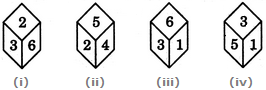In this question, you can see that in figure 1 and 2, 2 and 6 are adjacent to the number 3. While in figure 2, 3 is nowhere mentioned. In figure 3 also you will find that the 1 and 6 appear adjacent to 3. Thus from the figure 1, 2, and 3, you will find that 1, 2, 5, and 6 are adjacent to 3. From the options, the only number opposite to 3 is 4. Thus option (2) is the correct answer.

### Example 3

Q. From the given figure find the cube that will best represent after folding the paper.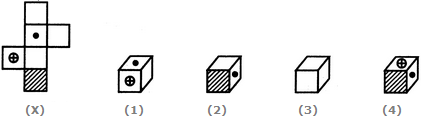1. Only 1                           2. Only 2

3. All of them                  4. None of them

In this question when you fold the paper to form a cube, then the square with the line will be opposite to the one with the dot. Another square which has a circle symbol on it with the plus sign in it will lie opposite to the blank face. The remaining two blank faces will be opposite to each other. Figure 2 and 4 are not possible since both of them have shaded(lines) part of the adjacent of the dot. While figure 3 shows all the blank faces which means that the remaining three faces have some sort of symbols on them. This means that they are adjacent to each other which is also not possible. Thus only one figure out of the given figures is possible and that is why option (1) is the correct answer.

## Practice Questions

Q. Identify the correct figure from the options.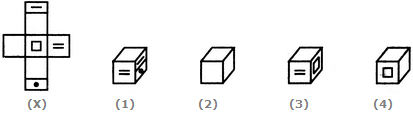A. 1, 2, and 3                     B. Only 1

C. 1, 2, 3, and 4                D. 1 and 3

Q. In the figure different positions of X, Y and Z is given. Which is the number at the bottom of the figure Y? (26)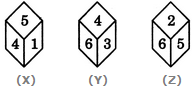A. 2                                      B. 3

C. 5                                      D. Cannot be determined

Q. What is the correct figure that will be when the piece of the paper is folded to make a cube? (7)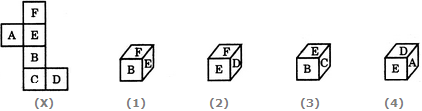A. 2 only                             B. 3 only

C. 4 only                             D. 1, 2, and 3

Share with friends

## Customize your course in 30 seconds

##### Which class are you in?
5th
6th
7th
8th
9th
10th
11th
12th
Get ready for all-new Live Classes!
Now learn Live with India's best teachers. Join courses with the best schedule and enjoy fun and interactive classes.Ashhar Firdausi
IIT Roorkee
BiologyDr. Nazma Shaik
VTU
ChemistryGaurav Tiwari
APJAKTU
Physics
Get Started

## Browse

##### Cubes and Dices TestSubscribe
Notify of

## Question Mark?

Have a doubt at 3 am? Our experts are available 24x7. Connect with a tutor instantly and get your concepts cleared in less than 3 steps.